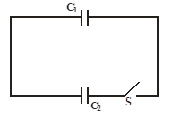Capacitance
Question

# Two capacitors ${\mathrm{C}}_{1}$ and ${\mathrm{C}}_{2}$ having capacitance 2 mF and 4 mF respectively are connected as shown in the figure. Initially ${\mathrm{C}}_{1}$ has charge 4 mC and ${\mathrm{C}}_{2}$ is uncharged. After long time closing the switch S :Easy
Solution

## Charge will flow till algebraic sum of potential difference across the capacitors is zero.

Get Instant Solutions